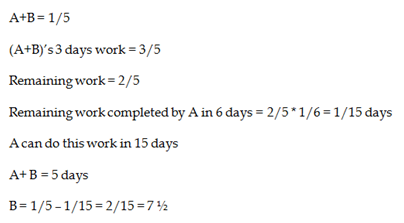# RRB ALP 2018 Practice Test Papers | Arithmetic Questions (Day-73)

Dear Aspirants, Here we have given the Important RRB ALP & Technicians Exam 2018 Practice Test Papers. Candidates those who are preparing for RRB ALP 2018 can practice these Arithmetic Questions to get more confidence to Crack RRB 2018 Examination.

[WpProQuiz 3021]

Click “Start Quiz” to attend these Questions and view Solutions

1) Atul had Rs. 2100 left after spending 70 % of the money he took for shopping. How much money did he have initially?

a) 4000

b) 5000

c) 6000

d) 7000

2) A shopkeeper bought 60 oranges and 40 bananas. He found 10% of Appleand 5% of Guava were rotten. Find the percentage of fruits in good condition.

a) 95%

b) 96 %

c) 92%

d) 91%

3) In an election, candidate A got 75% of the total  votes and the total numbers of votes is 64000,.. If 15% of the total votes of A were declared invalid  thenfind the number of valid vote polled in favour of candidate A.

a) 45000

b) 40200

c) 40800

d) 48000

4) What is the dividend, if divisor is 17, the quotient is 9 and the remainder is 5?

a) 150

b) 158

c) 140

d) 146

5) The inner circumference of a circular path around a circular lawn is 220 m. What is the radius of the outer circumference of the path, if the path is 15 m wide?

a) 45m

b) 50m

c) 55 m

d) 35m

6) 56%of x – 72% of 60 = 180.8

Find the value of x

a) 100

b) 200

c) 400

d) 500

7) In a Δ ABC, D and E are points on the sides AB and AC respectively such that DE BC.  If AD = 6 cm, DB = 9 cm and AE = 8 cm, Find AC.

a) 10

b) 20

c) 30

d) 40

8) Find the missing term in the series

72, 36, 54, x, 472.5, 2126.25

a) 172

b) 155

c) 140

d) 135

9) The average income of P, Q and R is Rs. 8,000 per month and the average income of Q, R and S is Rs. 7000 per month. If the salary of P is twice that of S, then the average salary of Q and R is
a) 5000
b) 7000
c) 10500
d) 9000

10) A and B together can complete a work in 5 days. They start together. But, after 3 days, B left the work. If the remaining work is completed 6 more days, B alone could do the work in

a) 10 days

b) 7 ½ days

c) 12 days

d) 6 days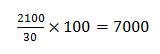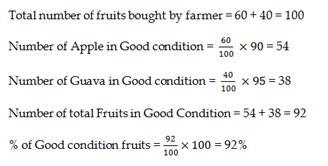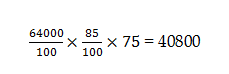Dividend = divisor × Quotient + Remainder

D = 17 × 9 + 5

= 153 + 5

D = 158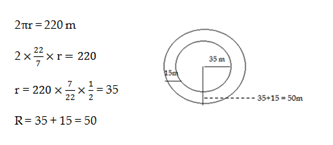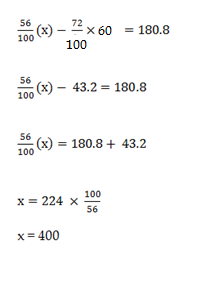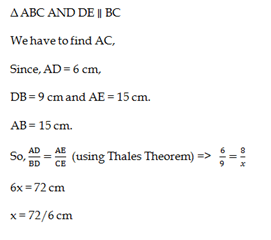72× 0.5 = 36

36× 1.5 = 54

54×2.5 = 135

135×3.5 = 472.5

472.5×4.5 = 2126.25

Total salary of PQR = 8000×3 = 24000

Total salary of QRS = 7000 × 3 = 21000
(P+Q+R) – (Q+R+S) = 24000 – 21000
P – S = 3000
2x – x = 3000
X = 3000 => S
2x = 6000 => P
P+Q+R = 24000
6000 + Q + R = 24000
Q + R = 18000
Average of Q+R = 18000/2 = 9000